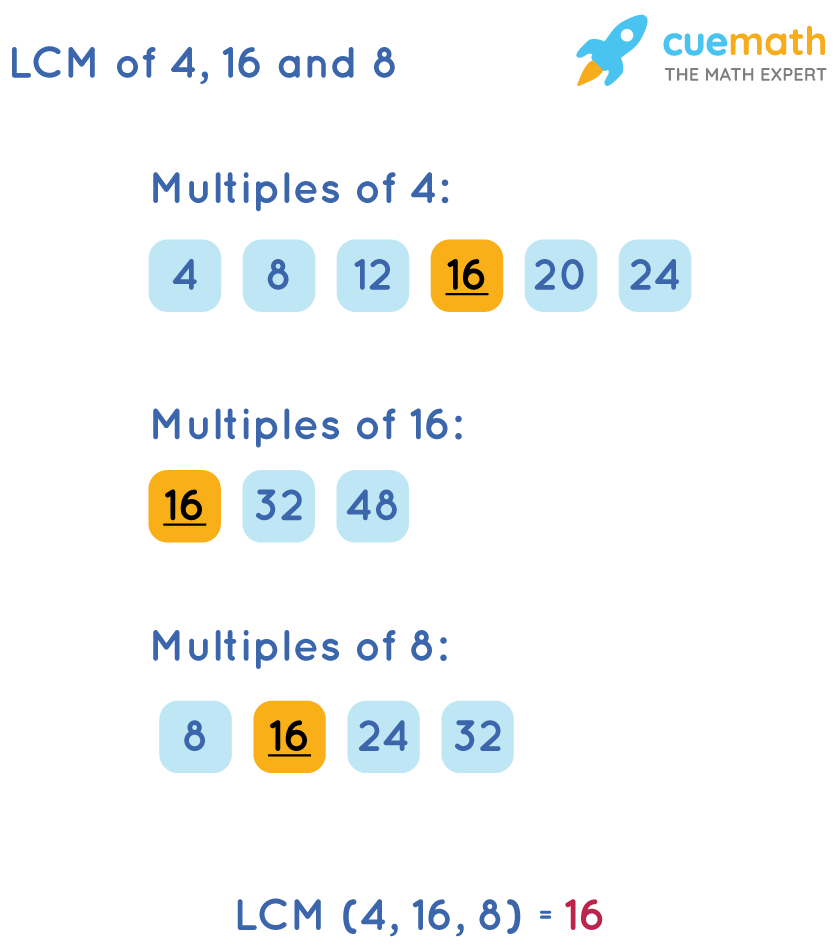# Express the fractions 3/4, 7/16, and 5/8 with the lcd.

## Question: <!MMtd@{border:@1px@solid@Cccc;}br@{mso-data-placement:same-cell;}MM> Express the fractions 3/4, 7/16, and 5/8 with an LCD.

LCD is the least common denominator.

## Answer: 12/16, 7/16, and 10/16

We will find the LCM of 4, 16 and 8 to find the LCD

## Explanation:

We will list the first few multiples of 4, 16 and 8 and then identify the least among those multiples. The least common multiple will be the least common divisor of the given fractions.So, the common divisor of 4, 16 and 8 is 16

3/4 = 12/16, 5/8 = 10/16